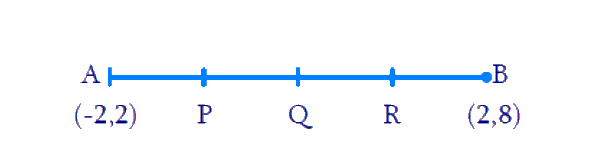# Ex.7.2 Q9 Coordinate Geometry Solution - NCERT Maths Class 10

Go back to  'Ex.7.2'

## Question

Find the coordinates of the points which divide the line segment joining $$A (-2, 2)$$ and $$B(2, 8)$$ into four equal parts.

Video Solution
Coordinate Geometry
Ex 7.2 | Question 9

## Text Solution

Reasoning:

The coordinates of the point $$P(x, y)$$ which divides the line segment joining the points $$A(x_1, y_1)$$ and $$B(x_2, y_2)$$, internally, in the ratio $$m_1 : m_2$$ is given by the Section Formula.

What is the known?

The $$x$$ and $$y$$ co-ordinates of the points $$A$$ and $$B$$.

What is the unknown?

The coordinates of the points which divide the line segment joining $$A \;(-2, 2)$$ and $$B\;(2, 8)$$ into four equal parts.

Steps:From the Figure,

By observation, that points $$P$$, $$Q$$, $$R$$ divides the line segment $$A(-2, 2)$$ and $$B(2, 8)$$ into four equal parts

Point $$P$$ divides the line segment $$AQ$$ into two equal parts

Hence, Coordinates of

\begin{align}\!\! P \!&\!=\!\! \!\left[ \!{\frac{{\!1\! \times \!2 \!+\! 3 \!\times \!( - 2)}}{{1 + 3}}\!,\!\frac{{1 \times \!8\! + \!3\! \times\!2}}{{1 + 3}}}\!\! \right]\!\! \\&= \left[ { - 1,\;\frac{7}{2}} \right]\end{align}

Point $$Q$$ divides the line segment $$AB$$ into two equal parts

Coordinates of

\begin{align} Q &= \left[ {\frac{{2 + ( - 2)}}{2},\;\frac{{2 + 8}}{2}} \right]\\ &= (0,\;5)\end{align}

Point $$R$$ divides the line segment $$BQ$$ into two equal parts

Coordinates of

\begin{align}\!\! R &\!=\!\left[\! {\frac{{3\! \times\! 2\! + \!1 \!\times\! ( -\! 2)}}{{3 + 1}}\!,\!\frac{{3\! \times\! 8\! + \!1\! \times\! 2}}{{3 + 1}}}\! \right]\;\\ &=\left[1, \frac{13}{2}\right]\end{align}

Learn from the best math teachers and top your exams

• Live one on one classroom and doubt clearing
• Practice worksheets in and after class for conceptual clarity
• Personalized curriculum to keep up with school